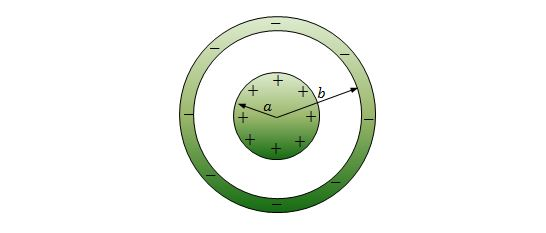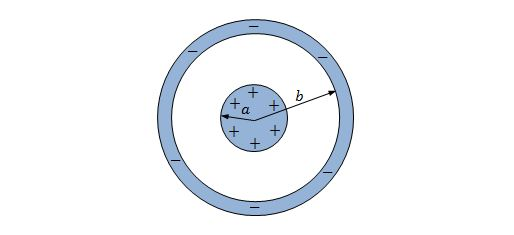Electricity and Magnetism

Gauss' law to find capacitanceThe concentric plates of a spherical capacitor have radii $a=4.1\text{ mm}$ and $b=4.6\text{ mm}.$ The above diagram shows the cross sectional feature of the capacitor. What is the approximate capacitance of the capacitor?

The value of the permittivity constant is $\varepsilon_0=8.85 \times 10^{-12} \text{ F/m}.$

What is the approximate capacitance of a copper sphere with a radius of $2.50\text{ mm}?$

The value of the permittivity constant is $\varepsilon_0=8.85 \times 10^{-12} \text{ F/m}.$A cylindrical capacitor has radii $a=1.1\text{ mm}$ and $b=2.9\text{ mm}$ and length $L=6.0\text{ cm},$ as shown in the above cross sectional feature of the capacitor. What is the approximate capacitance of the capacitor?

The value of the permittivity constant is $\varepsilon_0=8.85 \times 10^{-12} \text{ F/m}.$

Suppose that $4$ identical mercury spheres with radius $R=1.20\text{ mm}$ are merged to one sphere. What is the approximate capacitance of the merged mercury drop?

The value of the permittivity constant is $\varepsilon_0=8.85 \times 10^{-12} \text{ F/m}.$

A parallel-plate capacitor has $58.0\text{ cm}^2$ of plate area and $1.20\text{ mm}$ separation. What is the approximate capacitance of the capacitor?

The value of the permittivity constant is $\varepsilon_0=8.85 \times 10^{-12} \text{ F/m}.$

×